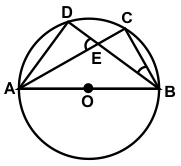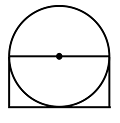# CREST Mathematics Olympiad Class 8 Previous Year Papers

## Syllabus:

Section 1: Rational Numbers, Squares and Square Roots, Cubes and Cube Roots, Exponents and Powers, Comparing Quantities, Algebraic Expressions and Identities, Linear Equations in One Variable, Understanding Quadrilaterals, Constructions, Mensuration, Visualizing Solid Shapes, Data Handling, Direct and Inverse Variations, Factorization, Introduction to Graphs, Playing with Numbers.

Achievers Section: Higher Order Thinking Questions - Syllabus as per Section 1

 Q.1 Kenster took some loan at 6% per annum simple interest during the first year with an increase of 0.5% for each year. After 4 years she paid \$3,375 as interest. How much was the loan?
 Q.2 A sum of money amounts to \$9800 after 5 years and \$12005 after 8 years at the same rate of simple interest. Find the rate of interest per annum:
 Q.3 A boy is running at a speed of p km/h to cover a distance of 1 km. But, due to slippery ground, his speed is reduced by q km/h (p > q). If he takes r hours to cover the distance, then which of the following is the correct relation between time, speed and distance?
 Q.4 If 2x-1 + 2x+1 = 320, then find the value of x:
 Q.5 If the angles of a triangle are (x - 35)°, (x - 25)° and (1/2x - 10)°, then find the value of x:
 Q.6 Multiply (3x3 - 7x + 8 - 2x2) by (4x2 + 6):
 Q.7 In the given figure, O is the centre of the circle, ∠CBE = 25⁰ and  ∠DEA = 60⁰. Find the measure of ∠ADB:Q.8 If AB = 5cm, BC = 4 cm, ∠B= 90° and opposite sides are equal, then which of the following figure can be constructed?
 Q.9 Resolve into factors: (a - b)2 - (a - b)3
 Q.10 The area of the circle is 616 cm2. What is the area of the rectangle?Answers to Previous Year Questions from CREST Olympiads:

 Q.1 a Q.2 c Q.3 a Q.4 d Q.5 d Q.6 b Q.7 c Q.8 b Q.9 a Q.10 c

Previous Year PDF of CREST Mathematics Olympiad for Class 8: